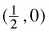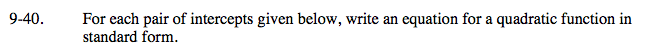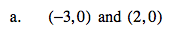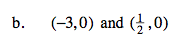Home > A2C > Chapter 9 > Lesson 9.1.2 > Problem9-40

9-40.
1. For each pair of intercepts given below, write an equation for a quadratic function in standard form. Homework Help ✎

1. (−3, 0) and (2, 0)

2. (−3, 0) andUse the Zero Product Property to write an equation in factored form and then simplify.

y = (x + 3)(x − 2)
y = x2 + x − 6See part (a).